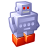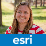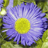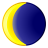# Calculate Geometry in Model Builder

32900
20
08-19-2011 01:43 PMOccasional Contributor II
I am looking for an easy method, similar to how you do it in ArcMap, to calculate geometry for two fields.  I want to add the lat and long to a point feature class that I have.  The coordinate system of the point feature class is not in geographic coordinates.  Any ideas.

Casey
Tags (3)
20 RepliesbyEsri Contributor
You can use the Add XY Coordinates tool to expose the xy coordinates of your point data. See this link http://http://help.arcgis.com/en/arcgisdesktop/10.0/help/index.html#//001700000032000000.htm

You should be able to use this in a model. However, before you do this you would need to re-project the data into a geographic coordinate system like WGS1984 or NAD1983 to get the values in decimal degrees. You would run the tool on this new feature class and that is where the information would be put. You can then do a join on data that is in the projected coordinate system to get its long/lats in decimal degrees.

Also in the model, you can also use Add Field then the Calculate Field tools. In the Calculate Field tool, use the following python expression.

```def find(shape):
point = shape.getPart(0)
return point.X```

`find(!Shape!)`

(sub point.Y to get the Y coordinates)

This will however use the coordinate system of the data so again you may need to re-project the data before you use the Calculate Field tool. Again, you can join the data back to your projected coordinate dataset.Occasional Contributor II
Thank you Stephanie, I used the add field and calculate field option.

CaseybyEsri Contributor
Great! Glad to hear that worked good for you! :cool:New Contributor II
Hello:
I saw this post, and since it related to my quesiton, I decided to add here.  I am basically trying to calculate geometry for two fields using model builder.  The first field is predefined as 'Total Miles' to house total mileage for all stream segments in that field.  The second is 'Need miles' that will house mileage for a fraction of the records.  I'll determine what records using select by attributes, then calculate geometry (mileage).  My final predefined field is the percentage of Need Miles to the Total Miles, which I can derive using Calculate field in model builder.  I know, too much information.  Basically, I just need to know how to Calculate Geometry in model builder?  I can't seem to locate the function in stand-alone format to drag in to model builder, and assume there is another approach, most likely a simple one.  Thanks in advance!
MarkOccasional Contributor
If you are looking to calculate the geometry of a shape and then put that value in a field, then you can do with python. Look at the ArcGIS Desktop Help under 'Calculate Field Examples', towards to the bottom it shows how to calculate geometry. For example

Area:  !Shape.Area!
Length: !Shape.Length!
X/Y/Z/M for first point in a line !Shape.firstPoint.X!

If you are looking to convert units (e.g. KM to Miles) you can even do that within the expression.

So drag in the 'Calculate Field' tool to Modelbuilder, and then build the expression to calculate the geometry you need. (Note I'm assuming your using 10.0+, I don't think this Python capability was in pre 10)New Contributor II
So, using the calculate field function in Model Builder.  I just need to my emplty field to equal !Shape.Length!?  How does it know the calculation is set to miles?  Thanks for your time!New Contributor II
I ran a test run, and although the fields are populated, their units aren't in miles.  I must be missing something simple...  I am using Arc10, and I have converted to an appropriate equal distance projection.  Regards...byMVP Honored Contributor
I ran a test run, and although the fields are populated, their units aren't in miles.  I must be missing something simple...  I am using Arc10, and I have converted to an appropriate equal distance projection.  Regards...

I am not sure how much influence the dataframe has on the field calculator.  I suspect it only uses the native projection and units of the feature class when you just put !Shape.Length!.

If you are OK with the original projection of the feature class, at 10 I believe if you choose the Python 9.3 option in the Model Builder Field Calculator tool you can adjust units by using:

!Shape.Length@Miles! # or substitute any other appropriate units for Length.

I am not sure how to emulate the geometry calculator's ability to apply the dataframe projection to feature classes that were stored using a different projection through the field calculator (At least not in Python.  At 9.3 VBA would do it, but not at 10 using VB script).New Contributor II
Perfect, I originally didn't add the @mile.  Thanks Richard for your time!